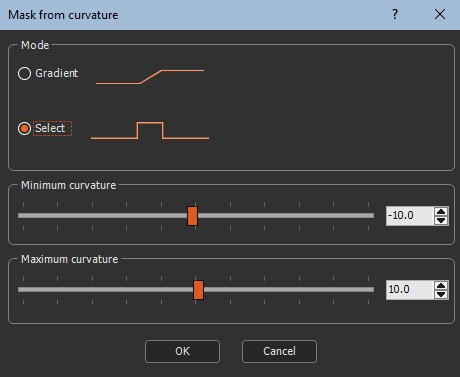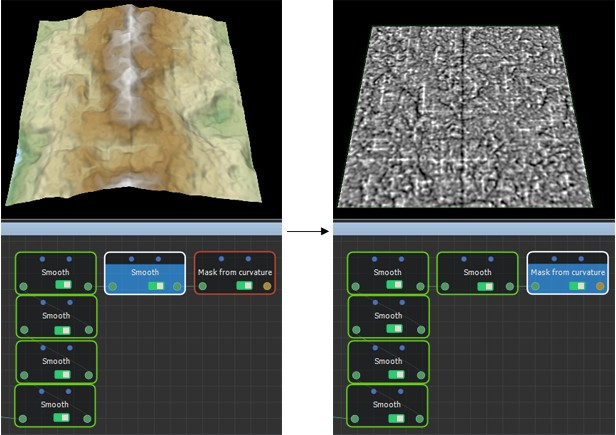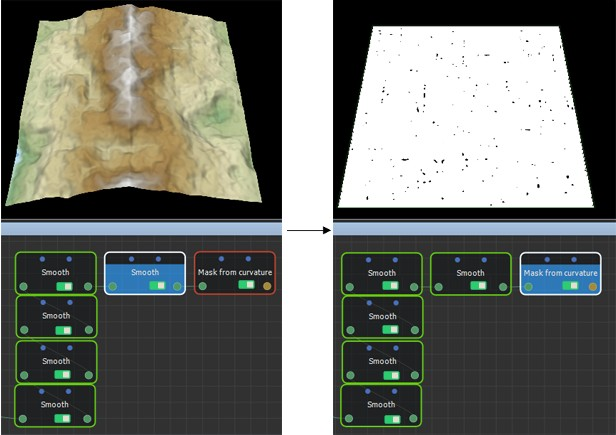This node creates a mask from the curvature of the terrain. The computed mean curvature corresponds to the average of the two principal curvatures. High curvature points, or positive values, represent the concave parts of the terrain (depressions) and low curvature points, or negative values, represent the convex parts of the terrain (projections). Zero curvature represents parts of the surface where the slope is constant (planes or a constant rise).

By default, the curvature is computed for the smallest details, but it can also be used to detect large concavities and convexities. To do so, the curvature is computed on a smoother terrain that contains only the low frequencies of the surface: you can construct such a terrain by using at least two chained smooth nodes with their strength equal to 1 and their radius proportional to the size of the features you want to extract.

To add a Mask from curvature node, right-click in the Graph Editor and select Create Node  > Mask Generation  > Mask from curvature.Double click on the node to open its parameters:### Editing a Mask from curvature node

Two modes exist to edit the Mask from curvature node.

• Gradient mode: In this mode, a gradient of values exists, with 0 corresponding to the defined Minimum curvature and 1 corresponding to the defined Maximum curvature. Use the sliders to adjust the values.

At each vertex, the mask has a value of 0 (black color) if the curvature of the terrain is less than or equal to the minimum curvature; it has a value of 1 (white color) if the curvature of the terrain is higher or equal to the maximum curvature; it has a value between 0 and 1 if the curvature of the terrain lies between these two curvatures.• Select mode: At each vertex, the mask has a value of 1 (white color) if the curvature of the terrain is between the Maximum curvature and the Minimum curvature; otherwise, the mask is set to 0 (black color). Set the range using the sliders.Convexity and curvature are two closely related terms. This node differs from the Mask from convexity node in the sense that it provides the convexity or curvature at each of the vertices individually, whereas the Mask from convexity node gives information on a much larger scale (the convexity or the curvature calculated on a very large number of vertices).

### Parameters

Parameter Use
Gradient mode The mask has a gradient with values between 0 for the minimum curvature up to 1 for the maximum curvature.
Select mode The mask is set to 1 for curvatures between the minimum curvature and maximum curvature; otherwise it is set to 0.
Maximum curvature The maximum curvature of the terrain.
Minimum curvature The minimum curvature of the terrain.